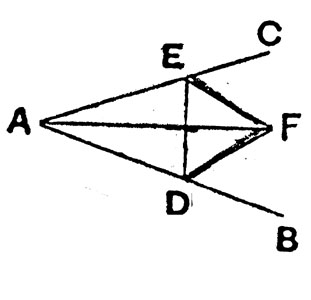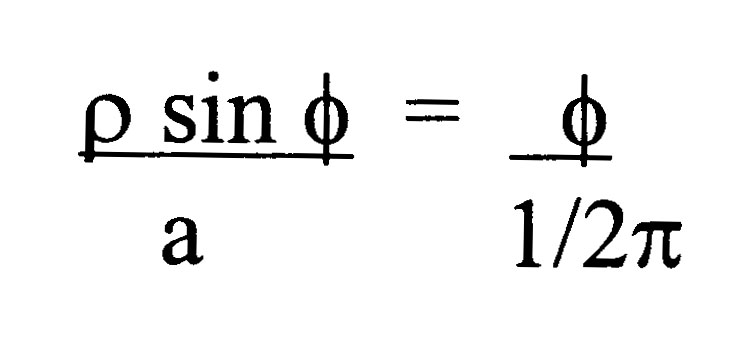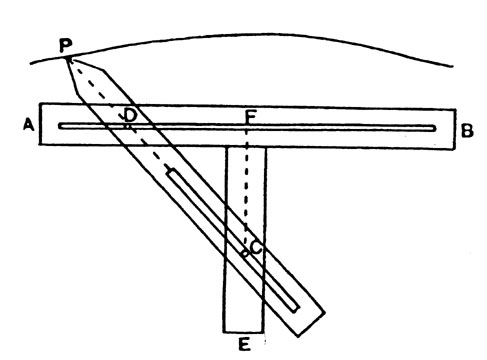## The Problem of Angle Trisection in Antiquity

### A. JackterHistory of MathematicsRutgers, Spring 2000

The problem of trisecting an angle was posed by the Greeks in antiquity. For centuries mathematicians sought a Euclidean construction, using "ruler and compass" methods, as well as taking a number of other approaches: exact solutions by means of auxiliary curves, and approximate solutions by Euclidean methods. The most influential mathematicians to take up the problem were the Greeks Hippias, Archimedes, and Nicomedes. The early work on this problem exhibits every imaginable grade of skill, ranging from the most futile attempts, to excellent approximate solutions, as well as ingenious solutions by the use of "higher" curves [Hobson]. Mathematicians eventually came to the empirical conclusion that this problem could not be solved via purely Euclidean constructions, but this raised a deeper problem: the need for a proof of its impossibility under the stated restriction.

According to a traditional classification used in antiquity there are three types of problems in geometry: plane, solid, and linear [Thomas]. `Plane' problems are those which can be solved by "Euclidean" methods, using straight lines and circumferences of circles. Using one or more of the sections of the cone, called conic surfaces, one solves 'Solid' problems. Problems involving other lines besides those mentioned above, having a less natural and more complicated origin because they are generated from more irregular surfaces or by purely mechanical constructions, are called `linear' [Thomas]. The early mathematicians were particularly interested in trisecting angles by `plane' methods. As it turns out, the trisection of an angle is not a `plane' problem, but a `solid' one [Heath]. This is why these early mathematicians failed to find a general construction for angle trisection using Euclidean constructions, though they did find elegant solutions based on the use of conic sections [Heath] and other more sophisticated curves.

The trisection of an angle, or, more generally, dividing an angle into any number of equal parts, is a natural extension of the problem of the bisection of an angle, which was solved in ancient times. Euclid's solution to the problem of angle bisection, as given in his Elements, is as follows:"To bisect a given rectilineal angle: Let the angle BAC be the given rectilineal angle. Thus it is required to bisect it. Let a point D be taken at random on AB; let AE be cut off from AC equal to AD; let DE be joined, and on DE let the equilateral triangle DEF be constructed; let AF be joined. I say that the straight line AF has bisected the angle BAC. For, since AD is equal to AE, and AF is common, the two sides DA, AF are equal to the two sides EA, AF respectively. And the base DF is equal to the base EF; therefore the angle DAF is equal to the angle EAF. Therefore the given rectilineal angle BAC has been bisected by the straight line AF." [Euclid, p. 264] Note that Euclid's construction makes use of only lines and circles.

The earliest mathematician whose work bears on the problem of angle trisection was the Greek Hippias, who was born about 460 BC and died about 400 BC. Hippias' main contribution to Greek geometry was his invention of a curve called the quadratrix [D.E. Smith]. The quadratrix was used originally for the problem of squaring the circle, and later for the trisection of an angle [Smith,Euclid].As shown the figure above, the construction of the quadratrix is as follows: "Suppose that ABCD is a square and BED a quadrant of a circle with center A. Suppose
(1) that a radius of the circle moves uniformly about A from the position AB to the position A D, and
(2) that in the same time the line BC move uniformly, always parallel to itself, and with its extremity B moving along BA, from the position BC to the position AD"
[Euclid, p. 266]. "Then, in their ultimate positions, the moving straight line and th e moving radius will both coincide with AD; and at any previous instant during the motion the moving line and the moving radius will by their intersection determine a point, as F or L. The locus of these point is the quadratrix" [Heath, p. 227]. "The property of the curve is that, if F is any point, the arc BED is to the arc ED as AB is to FH" [Euclid, p. 267]. "In other words, if phi is the angle FAD made by any radius vector AF with AD, rho the length of AF, and a the length of the side of the square,"Now the angle EAD can not only be trisected but divided in any given ratio by means of the quadratrix" [Heath, p. 267]. "For let FH be divided at F' in the given ratio. Draw F'L parallel to AD to meet the curve in L: join AL, and produce it to meet the circle in N. Then the angles EAN, NAD are in the ratio of FF' to F'H. Thus the quadratrix lends itself quite readily to the division of any angle in a given ratio" [Heath, p. 227].

Another mathematician who contributed greatly to the development of ancient geometry was Archimedes, who was born in 287 BC in Syracuse, Sicily, and died there during the Roman conquest of Syracuse in 212 BC [Dijksterhuis, pp.9-10]. Archimedes worked in Sicily, but corresponded heavily with his mathematical colleagues, the Alexandrian scholars Eratosthenes, Conon, and Dositheus. He would send them statements of the propositions that he had proved, and waited to see if his colleagues could find a proof on their own, and would later send detailed proofs to his fellow mathematicians in Alexandria. [Dijksterhuis, pp.11-12].

Archimedes created a curve that he called the spiral, which was used to investigate the squaring the circle and the trisection of an angle. He defined the spiral in a letter to Dositheus as follows: If a straight line drawn in a plane revolves uniformly any num ber of times about a fixed extremity until it returns to its original position, and if, at the same time as the line revolves, a point moves uniformly along the straight line beginning at the fixed extremity; the point will describe a spiral in the plane [Archimedes, p. 154]. The spiral discovered by Archimedes was used for angle trisection and more generally to solve the problem of cutting an angle contained by straight lines in a given ratio. Archimedes used these spirals in most of his works, not just to attempt to solve the trisection of angles problem [Dijksterhuis, p. 134].

"A proposition leading to the reduction of the trisection of an angle to another `verging' is included in the collection of Lemmas (Liber Assumptorum) which has come to us under the name of Archimedes through the Arabic. Though the Lemmas cannot have be en written by Archimedes in their present form, because his name is quoted in them more than once, it is probable that some of them are of Archimedean origin, and especially is this the case with Prop. 8, since the 'verging' suggested by it is of very muc h the same kind as those the solution of which is assumed in the treatise On Spirals, Props. 5-8" [Heath, p. 241].

"The proposition is as follows: If AB be any chord of a circle with center O, and AB be produced to C so that BC is equal to the radius, and if CO meet the circle in C, E, then the arc AE will be equal to three times the arc BD. Draw the chord EF parallel to AB, and join OB, OF. Since BO = BC, angle BOC = angle BCO. Now angle COF = 2 angle OEF = 2 angle BCO, by parallels, = 2 angle BOC.[Heath , p. 241]

Therefore angle BOF = 3 angle BOD, and arc BF = arc AE = 3 arc BD" [Heath, pp. 240-241]. This proposition gives a method of reducing the trisection of any angle. Suppose that AE is the arc to be trisected, and that ED is the diameter through E of the circle o f which AE is an arc. In order to find an arc equal to one third of AE, draw a line ABC through A, meeting the circle again in B and ED produced in C, such that BC is equal to the radius of the circle [Archimedes,Heath].

Another Greek mathematician to study the trisection of an angle was Nicomedes. Nicomedes was born about 280 BC and died about 210 BC in Greece [Heath]. There is not much known about the life of Nicomedes [Thomas]. What is known has been inferred from mathematical criticisms of Eratosthenes' mathematical efforts and of Nicomedes' works. Nicomedes is best known for his invention of the curve known as the conchoid [Heath], which serves as the basis for an angle trisection.

Proclos was a mathematician and Neoplatonist philosopher who lived from 410 to 485 AD [Euclid, p. 29]. In his discussion of the trisection of an angle, he said, "Nicomedes trisected any rectilineal angle by means of the conchoidal curves, the construction, order and prope rties of which he handed down, being himself the discoverer of their peculiar character. Others have done the same thing by means of the quadrtrices of Hippias and Nicomedes...Others again, starting from the spirals of Archimedes, divided any given recti lineal angle in any given ratio" [Heath, p. 225]. This curve was originally called the cochloid by Pappus, a geometer from Alexandria who probably lived in the third century, and called the conchoid by Proclos [Heath].

"There were varieties of the cochloidal curves; Pappus speaks of the 'first', 'second', 'third', and 'fourth', observing that the 'first' was used for trisecting an angle and duplicating the cube, while the others were useful for other investigations" [Heath, p. 238]. It is the 'first' curve that is involved with the trisection of an angle. Nicomedes constructed this curve by means of a mechanical device, as follows:[Heath, p. 239]

AB is a ruler with a slot in it parallel to its length, FE a second ruler fixed at right angles to the first, with a peg C fixed in it. A third ruler PC pointed at P has a slot in it parallel to its length with fits the peg C. D is a fixed peg on PC in a straight line with the slot, and D can move freely along the slot in AB. If then the ruler PC moves so that the peg D describes the length of the slot in AB on each side of F, the extremity P of the ruler describes the curve which is called a conchoid or cochloid. Nicomedes called the straight line AB the ruler, the fixed point C the pole, and the constant length PD the distance" [Heath, pp. 238-239].

"The fundamental property of the curve, which in polar coordinates would now be denoted by the equation r = a + b sec(theta), is that if any radius vector be drawn from C to the curve, as CP, the length intercepted on the radius vector between the curve and the straight line AB is constant" [Heath, p. 239]. "Proclos says that Nicomedes gave the construction, the order, and t he properties of the conchoidal lines; but nothing of his treatise has come down to us except the construction of the 'first' conchoid, its fundamental property, and the fact that the curve has the ruler or base as an asymptote in each direction. The dis tinction, however, drawn by Pappus between the "first', 'second', 'third' and 'fourth' conchoids may well have been taken from the original treatise, directly or indirectly" [Heath, pp. 239-240].

Carl Friedrich Gauss was born in 1777 and died in 1855 and is considered "the real founder of modern German mathematics" [Smith, p. 502]. Gauss realized that using only lines and circles, one could not solve the problems of trisecting an angle, nor that of duplicating a cube. Unfortunately, Gauss left no proof of either of these statements. However, he gave the construction of a regular 17-gon using only Euclidean constructions [Dunham]. Mathematicians were interested in determining whether the trisection of any angle was possible using only these methods. The first person to prove its impossibility results was Pierre Laurent Wantzel, who published his proofs in a paper called "Research on the Means of Knowing If a Problem of Geometry Can Be Solved with Compass and Straight-edge", in 1837 [Dunham, pp. 245-247]. In Wantzel's paper he proved the impossibility of the solution under Euclidean restrictions. Wantzel regarded the magnitudes involved not as geometric segments, but as numerical lengths, via analytic geometry. This let him use algebra and arithmetic rather than pure geometry [Dunham, p. 245].

Throughout history the problem of trisecting an angle, or more generally of cutting an angle into a given ratio, has challenged mathematicians. This quest endured for centuries. In ancient times Hippias, Archimedes, and Nicomedes all invented curves which suitable for ad hoc angle trisections, the quadratrix, the spiral, and the conchoid, but these curves did not provide the desired "Euclidean" construction for the trisection of angles, using only lines and circles. Nonetheless they were still valuable and ingenious additions to the field of geometry. The recognition that it was possible to give a proof of impossibility as precise and rigorous as a conventional mathematical solution represents a conceptual turning point for mathematics, and a satisfactory solution of this classical problem was not achieved until the 19th century.

### References

1. Archimedes, The Works of Archimedes, translated and edited by Sir T. L. Heath, Dover Publications, New York, 1953.
2. Clagett, M., Archimeds in the Middle Ages, vol. 1, University of Wisconsin Press, Madison, WI 1954.
3. Dijksterhuis, E. J., Archimedes, Princeton University Press, Princeton, NJ, 1987.
4. Dunham, W. The Mathematical Universe, John Wiley & Sons, Inc., New York, 1994.
5. Euclid, The Thirteen Books of Euclid's Elements, tras. by Sir T. Heath, Dover Publications, New York, 1956.
6. Eves, H. W., Mathematical Circles Squared, Prindle, Weber, and Schmidt, Boston, MA, 1972.
7. Gray, J., The Symbolic Universe, University Press, Oxford, 1999.
8. Heath, Sir T. L., A History of Greek Mathematics. Vol. 1: From Thales to Euclid, Dover Publications, New York, 1981.
9. Hobson, E. W., Squaring the Circle, Cambridge University Press, Cambridge, 1913.
10. Proclos, A Commentary on the First Book of Euclid's Elements, translated and edited by Morrow, Princeton University Press, Princeton, NJ 1970.
11. Roche, J. J., The Mathematics of Measurement: A Critical History, The Athlone Press, London, 1998.
12. Smith, D. E., History of Mathematics, vol. 1, Dover Publications, New York, 1923.
13. Tarrant, D., The Hippias Major, Arno Press, New York, 1976.
14. Thomas, I., Selections Illustrating the History of Greek Mathematics, William Heinemann, London, and Harvard University Press, Cambridge, MA, 1939, 1941.